Next: Model selection in ensemble Up: No Title Previous: Approximations of posterior pdf

# Ensemble learning

Ensemble learning, , is a recently introduced method for parametric approximation of the posterior distributions where Kullback-Leibler information, , , is used to measure the misfit between the actual posterior distribution and its approximation. Let us denote the observed variables by x and the unknown variables by y. The true posterior distribution p(y|x) is approximated with the distribution q(y|x)by minimising the Kullback-Leibler information.

 D(q(y | x) || p(y | x)) =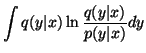=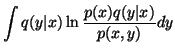=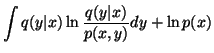(4)

The Kullback-Leibler information is greater than or equal to zero, with equality if and only if the two distributions, p(y|x) and q(y|x), are equivalent. Therefore the Kullback-Leibler information acts as a distance measure between the two distributions.

If we note that the term p(x) is a constant over all the models, we can define a cost function Cy(x) which we are required to minimise to obtain the optimum approximating distribution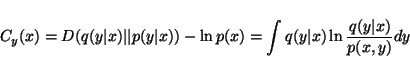(5)

We shall adopt the notation that the subindex of C denotes the variables that are marginalised over in the cost function. In general, they are the unknown variables of the model. The notation also makes explicit that the cost function Cy(x) gives an upper bound for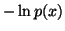. Here we use the same notation as with probability distributions, that is Cy(x | z) means
 Cy(x | z) =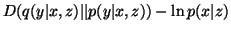=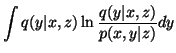(6)

and thus yields the upper bound for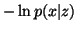.

Ensemble learning is practical if the terms p(x, y) and q(y | x)of the cost function Cy(x)can be factorised into simple terms. If this is the case, the logarithms in the cost function split into sums of many simple terms. By virtue of the definition of the models, the likelihood and priors are both likely to be products of simpler distributions, p(x, y) typically factorises into simple terms. In order to simplify the approximating ensemble, q is also modelled as a product of simple terms.

The Kullback-Leibler information is a global measure, providing that the approximating distribution is a global distribution. Therefore the measure will be sensitive to probability mass in the true posterior distribution rather than the absolute value of the distribution itself.

Training the approximating ensemble can be done by assuming a fixed parametric form for the ensemble (for instance assuming a product of Gaussians). The parameters of the distributions can then be set to minimise the cost function.

An alternative method is to only assume a separable form for the approximating ensemble. The distributions themselves can then be found by performing a functional minimisation of the cost function with respect to each distribution in the ensemble. While this method must always give ensembles with equivalent or lower misfits than those obtained by a assuming a parametric form, the distributions that are obtained are not always tractable and so the fixed form method may be more useful.Next: Model selection in ensemble Up: No Title Previous: Approximations of posterior pdf
Harri Lappalainen
2000-03-03